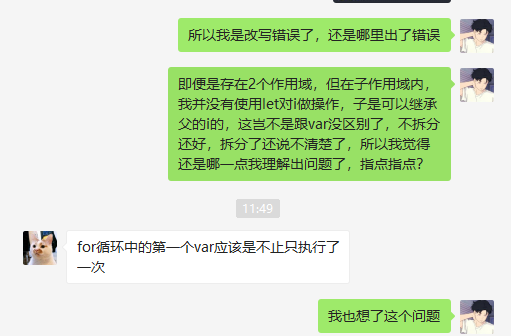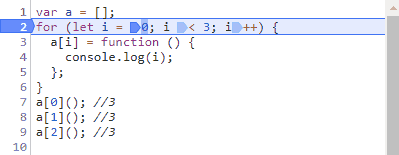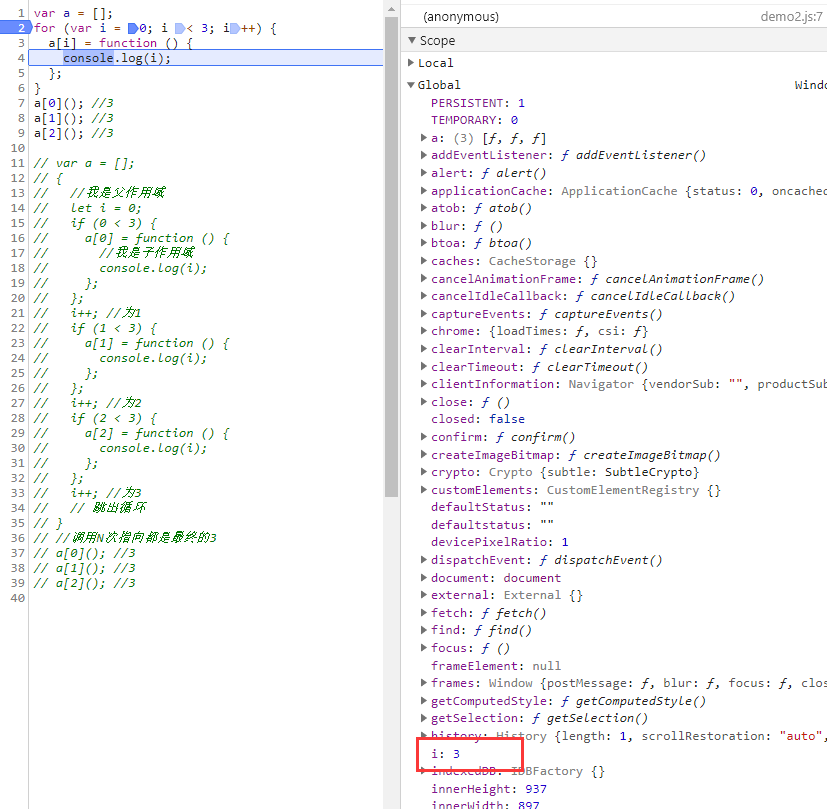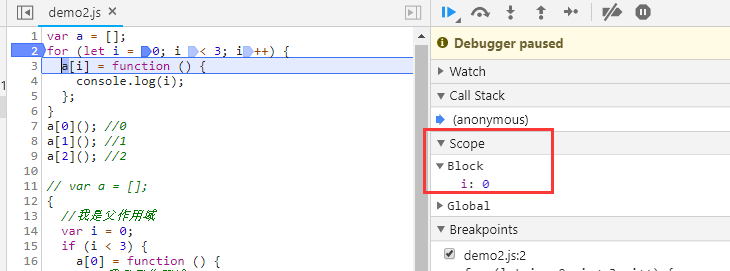# 听风是风

## for循环中let与var的区别，块级作用域如何产生与迭代中变量i如何记忆上一步的猜想一、一个简单的for循环问题与我思考后产生的问题

//使用var声明，得到3个3
var a = [];
for (var i = 0; i < 3; i++) {
a[i] = function () {
console.log(i);
};
}
a(); //3
a(); //3
a(); //3
//使用let声明，得到0,1,2
var a = [];
for (let i = 0; i < 3; i++) {
a[i] = function () {
console.log(i);
};
}
a(); //0
a(); //1
a(); //2

for循序的执行顺序是这样的：设置循环变量(var i = 0)  ==》循环判断(i<3)  ==》满足执行循环体 ==》循环变量自增(i++)

{
//我是父作用域
var i = 0;
if (0 < 3) {
a = function () {
//我是子作用域
console.log(i);
};
};
i++; //为1
if (1 < 3) {
a = function () {
console.log(i);
};
};
i++; //为2
if (2 < 3) {
a = function () {
console.log(i);
};
};
i++; //为3
// 跳出循环
}
//调用N次指向都是最终的3
a(); //3
a(); //3
a(); //3JavaScript 引擎内部会记住上一轮循环的值，初始化本轮的变量i时，就在上一轮循环的基础上进行计算。

那这就是JS引擎底层实现的问题了，我还真没法用自己的代码去模拟去实现，我们分别截图var与let断点情况下作用域的分布。PS：2019.4.19更新

var a = []; {
//我是父作用域
let i = 0;
if (i < 3) {
//这一步模拟底层实现
let k = i;
a[k] = function () {
//我是子作用域
console.log(k);
};
};
i++; //为1
if (i < 3) {
let k = i;
a[k] = function () {
console.log(k);
};
};
i++; //为2
if (i < 3) {
let k = i;
a[k] = function () {
console.log(k);
};
};
i++; //为3
// 跳出循环
}
a(); //0
a(); //1
a(); //2

PS：2019.11.28更新

var a = []
for (let y = {i: 0}; y.i < 3; y.i++) {
a[y.i] = function () {
console.log(y.i);
};
};
a();
a();
a();

var a = []; {
//我是父作用域
let y = {
i: 0
};
if (y.i < 3) {
//这一步模拟底层实现
let k = y;
a[k.i] = function () {
//我是子作用域
console.log(k.i);
};
};
y.i++; //为1
if (y.i < 3) {
let k = y;
a[k.i] = function () {
console.log(k.i);
};
};
y.i++; //为2
if (y.i < 3) {
let k = y;
a[k.i] = function () {
console.log(k.i);
};
};
y.i++; //为3
// 跳出循环
}
a(); //3
a(); //3
a(); //3

var a = []
var b = {i:0};
for (let y = b.i; y < 3; y++) {
a[y] = function () {
console.log(y);
};
};
a();
a();
a();

posted on 2019-03-23 18:31  听风是风  阅读(11450)  评论(41编辑  收藏  举报